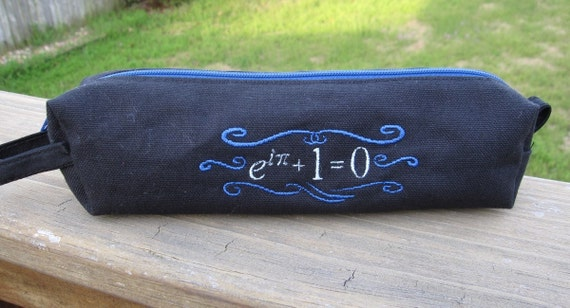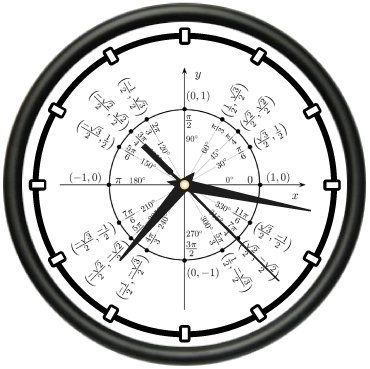# Thoughts on 1/7 and Other Rational Numbers: Index

I’m using the Twelve Days of Christmas (and perhaps a few extra days besides) to do something that I should have done a long time ago: collect past series of posts into a single, easy-to-reference post. The following posts formed my series the decimal expansions of rational numbers.

Part 1: A way to remember the decimal expansion of$\displaystyle \frac{1}{7}$.

Part 2: Long division and knowing for certain that digits will start repeating.

Part 3: Converting a repeating decimal into a fraction, using algebra.

Part 4: Converting a repeating decimal into a fraction, using infinite series.

Part 5: Quickly converting fractions of the form$\displaystyle \frac{M}{10^t}$,$\displaystyle \frac{M}{10^k-1}$, and$\displaystyle \frac{M}{10^t (10^k-1)}$ into decimals without using a calculator.

Part 6: Converting any rational number into one of the above three forms, and then converting into a decimal.

Part 7: Same as above, except using a binary (base-2) expansion instead of a decimal expansion.

Part 8: Why group theory relates to the length of the repeating block in a decimal expansion.

Part 9: A summary of the above ideas to find the full decimal expansion of$\displaystyle \frac{8}{17}$, which has a repeating block longer than the capacity of most calculators.

Part 10: More thoughts on$\displaystyle \frac{8}{17}$.

# Calculator Errors: When Close Isn’t Close Enough (Index)

I’m using the Twelve Days of Christmas (and perhaps a few extra days besides) to do something that I should have done a long time ago: collect past series of posts into a single, easy-to-reference post. The following posts formed my series on how I remind students about Taylor series. I often use this series in a class like Differential Equations, when Taylor series are needed but my class has simply forgotten about what a Taylor series is and why it’s important.

Part 1: Propagation of small numerical errors.

Part 2: A tragedy during the 1991 Gulf War that was a direct result of calculator rounding.

# Square roots and Logarithms Without a Calculator: Index

I’m using the Twelve Days of Christmas (and perhaps a few extra days besides) to do something that I should have done a long time ago: collect past series of posts into a single, easy-to-reference post. The following posts formed my series on computing square roots and logarithms without a calculator.

Part 1: Method #1: Trial and error.

Part 2: Method #2: An algorithm comparable to long division.

Part 3: Method #3: Introduction to logarithmic tables. At the time of this writing, this is the most viewed page on my blog.

Part 4: Finding antilogarithms with a table.

Part 5: Pedagogical and historical thoughts on log tables.

Part 6: Computation of square roots using a log table.

Part 7: Method #4: Slide rules

Part 8: Method #5: By hand, using a couple of known logarithms base 10, the change of base formula, and the Taylor approximation$ln(1+x) \approx x$.

Part 9: An in-class activity for getting students comfortable with logarithms when seen for the first time.

Part 10: Method #6: Mentally… anecdotes from Nobel Prize-winning physicist Richard P. Feynman and me.

Part 11: Method #7: Newton’s Method.

# Why does x^0 = 1 and x^(-n) = 1/x^n? (Index)

I’m using the Twelve Days of Christmas (and perhaps a few extra days besides) to do something that I should have done a long time ago: collect past series of posts into a single, easy-to-reference post. The following posts formed my series why the rules for$x^0$ and$x^{-n}$ work the way they do.

Part 1: Multiplication and division.

Part 2: The Laws of Exponents.

# Why does 0! = 1? (Index)

I’m using the Twelve Days of Christmas (and perhaps a few extra days besides) to do something that I should have done a long time ago: collect past series of posts into a single, easy-to-reference post. The following posts formed my series on how I explain to students that$0! = 1$.

Part 1: Multiplication and division.

Part 2: Combinatorics.

Now that Christmas is over, I can safely share the Christmas gifts that I gave to my family this year thanks to Nausicaa Distribution (https://www.etsy.com/shop/NausicaaDistribution):

Euler’s equation pencil pouch:Box-and-whisker snowflakes to hang on our Christmas tree:And, for me, a wonderfully and subtly punny “Confidence and Power” T-shirt.Thanks to FiveThirtyEight (see http://fivethirtyeight.com/features/the-fivethirtyeight-2014-holiday-gift-guide/) for pointing me in this direction.For the sake of completeness, here are the math-oriented gifts that I received for Christmas:# Arithmetic and Geometric Series: Index

I’m using the Twelve Days of Christmas (and perhaps a few extra days besides) to do something that I should have done a long time ago: collect past series of posts into a single, easy-to-reference post. The following posts formed my series on how I remind students about Taylor series. I often use this series in a class like Differential Equations, when Taylor series are needed but my class has simply forgotten about what a Taylor series is and why it’s important.

Part 1: Deriving the formulas for the$n$th term of arithmetic and geometric sequences.

Part 2: Pedagogical thoughts on conceptual barriers that students often face when encountering sequences and series.

Part 3: The story of how young Carl Frederich Gauss, at age 10, figured out how to add the integers from 1 to 100 in his head.

Part 4: Deriving the formula for an arithmetic series.

Part 5: Deriving the formula for an arithmetic series, using mathematical induction. Also, extensions to other series.

Part 6: Deriving the formula for an arithmetic series, using telescoping series. Also, extensions to other series.

Part 7: Pedagogical thoughts on assessing students’ depth of understanding the formula for an arithmetic series.

Part 8: Deriving the formula for a finite geometric series.

Part 9: Infinite geometric series and Xeno’s paradox.

Part 10: Deriving the formula for an infinite geometric series.

Part 11: Applications of infinite geometric series in future mathematics courses.

Part 12: Other commonly-arising infinite series.

# Why Does 0.999… = 1? (Index)

I’m using the Twelve Days of Christmas (and perhaps a few extra days besides) to do something that I should have done a long time ago: collect past series of posts into a single, easy-to-reference post. The following posts formed my series on different techniques that I’ll use to try to convince students that$0.999\dots = 1$.

Part 1: Converting the decimal expansion to a fraction, with algebra.

Part 2: Rewriting both sides of the equation$1 = 3 \times \displaystyle \frac{1}{3}$.

Part 3: Converting the decimal expansion to a fraction, using infinite series.

Part 4: A proof by contradiction: what number can possibly be between$0.999\dots$ and$1$?

Part 5: Same as Part 4, except by direct reasoning.